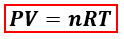# Problem: The pressure exerted by a gas on its container is directly proportional to ________.a. the volume of the containerb. the mass of the individual gas moleculesc. the Celsius temperature of the gasd. the number of molecules of gas in the samplee. the Fahrenheit temperature of the gas

###### FREE Expert Solution

Recall the ideal gas Law.P = pressure, atm
V = volume, L
n = moles, mol
R = gas constant = 0.08206 (L·atm)/(mol·K)
T = temperature, K

92% (448 ratings)###### Problem Details

The pressure exerted by a gas on its container is directly proportional to ________.

a. the volume of the container

b. the mass of the individual gas molecules

c. the Celsius temperature of the gas

d. the number of molecules of gas in the sample

e. the Fahrenheit temperature of the gas